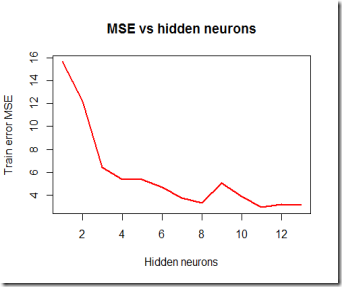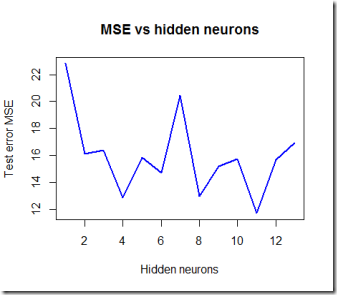Want to share your content on R-bloggers? click here if you have a blog, or here if you don't.

Recently I wrote a post for DataScience+ (which by the way is a great website for learning about R) explaining how to fit a neural network in R using the neuralnet package, however I glossed over the “how to choose the number of neurons in the hidden layer” part. The glossing over is mainly due to the fact that there is no fixed rule or suggested “best” rule for this task but the mainstream approach (as far as I know) is mostly a trial and error process starting from a set of rules of thumb and a heavy cross validating attitude.

As far as the number of hidden layers is concerned, at most 2 layers are sufficient for almost any application since one layer can approximate any kind of function. In this example I am going to use only  1 hidden layer but you can easily use 2. I suggest to use no more than 2 because it gets very computationally expensive very quickly. Furthermore more than 2 layers may get hard to train effectively.

### The rules of thumb

The most common rule of thumb is to choose a number of hidden neurons between 1 and the number of input variables. A slight variation of this rule suggests to choose a number of hidden neurons between one and the number of Inputs minus the number of outputs (assuming this number is greater than 1).  I see no reason to prefer say 12 neurons over 10 if your range of choices goes from say 1 to 18, therefore I decided to  use the cross validating approach and get the configuration that minimizes the test MSE while keeping an eye on over fitting and the train set error. Usually after a certain number of hidden neurons are added, the model will start over fitting your data and give bad estimates on the test set.

Here I am re-running some code I had handy (not in the most efficient way I should say) and tackling a regression problem, however we can easily apply the same concept to a classification task.

### Running the simulation

The basic idea to get the number of neurons right is to cross validate the model with different configurations and get the average MSE, then by plotting the average MSE vs the number of hidden neurons we can see which configurations are more effective at predicting the values of the test set and dig deeper into those configurations only, therefore possibly saving time too.

In order to do this I’m using a cross validating function that can handle the cross validating step in the for loop. Note that this code will take long to run (10 minutes), for sure it could be made more efficient by making some small amendments. Here is the code

It looks like the number of hidden neurons (with a single layer) in this example should be 11 since it minimizes the test MSE.  The red line is the training MSE and as expected goes down as more neurons are added to the model.
As you can see in the graphs below, the blue line which is the test MSE, starts to go up sharply after 11 possibly indicating over fitting. Four, eight and eleven hidden neurons are the configurations that could be used for further testing and better assessing crossvalidated MSE and predictive performance.Hope this was interesting.